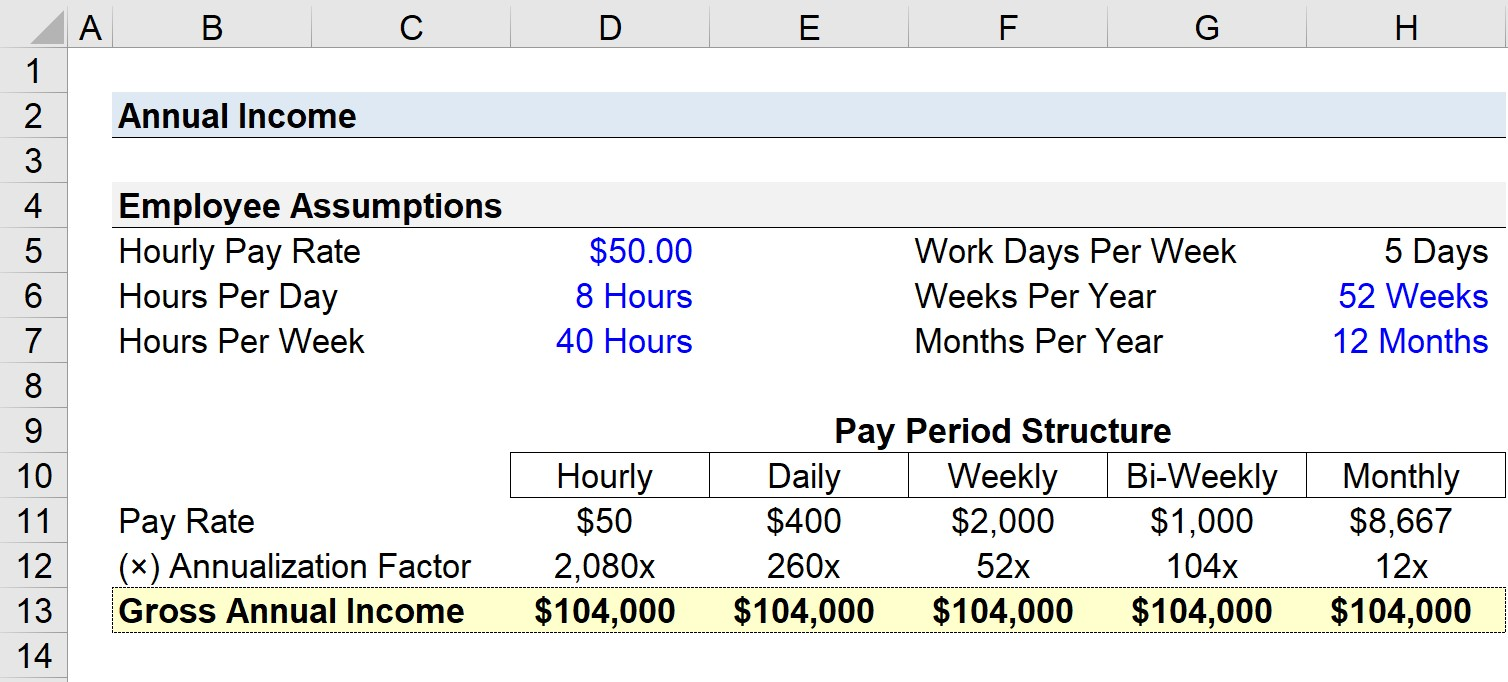# Annual Income

Guide to Understanding Gross Annual Income ("Yearly Salary")## How to Calculate Annual Income (Step-by-Step)

Before the net annual income can be estimated, calculating the gross annual income is the necessary first step.

From the perspective of an individual worker, gross income is the annual compensation before taxes and other deductions, i.e. the “top line” revenue of the employee.

The compensation of employees can be presented in various forms, so we’ll outline the formulas to convert a non-annual payment into an annualized figure.

A full-time worker would work an estimated 2,080 hours a year, assuming the employee works 40 hours a week for 52 weeks.

Of course, there is quite a bit of room for the actual figures to differ, but the annual income — especially for hourly compensation — is more so meant to be a rough approximation.

## Annualization Factor: Hourly, Daily, Weekly, Monthly Chart

### Full-Time Employee (FTE) Yearly Income Conversion

The chart below shows the annualization factors used for a standard full-time employee (FTE).

Of course, the implied annual income can be overstated (or understated) in reality because there could be sick days, company-wide days off, overtime, shift replacements, etc.

 Period Annualization Factor Hourly Rate 2,080x Daily Rate 250x Weekly Basis 52x Bi-Weekly Basis 104x Monthly Basis 12x

## Annual Income Formula (“Yearly Income”)

In order to calculate the total annual income, or “yearly income”, the pay rate for each pay period structure must be multiplied by the corresponding annualization factor.

Gross Annual Income = Periodic Pay Rate × Annualization Factor

The pay rate refers to the periodic amount of income earned in a specific time frame.

For instance, let’s say that an employee’s hourly pay rate is \$20.00. If the employee works 40 hours per week, the periodic pay rate on a weekly basis is \$800, i.e. the employee makes \$800 per week before any deductions such as taxes.

• Hourly Pay Rate = \$20.00
• Hours Per Week = 40 Hours
• Weekly Pay Rate = \$20.00 × 40 Hours = \$800

In order to annualize the employee’s weekly pay, we must multiply it by an annualization factor of 52x, which comes out to \$41.6k per year in gross annual income.

• Gross Annual Income = \$800.00 × 52x = \$41,600

The employee would have to then reduce their gross annual income by taxes owed and other deductions to arrive at net annual income.

## Annual Income Calculator — Excel Model Template

We’ll now move to a modeling exercise, which you can access by filling out the form below.Submitting ...

## Step 1. Full-Time Employee (FTE) Assumptions

Suppose a standard full-time employee (FTE) employee’s salary is paid at an hourly pay rate of \$50.00 per hour.

For our exercise, we’ll use the following assumptions regarding our hypothetical employee to estimate the pay rate and annualization factor under various scenarios.

• Hourly Pay Rate → \$50.00
• Hours Per Day → 8 Hours
• Hours Per Week → 40 Hours
• Work Days Per Week → 5 Days
• Weeks Per Year → 52 Weeks
• Months Per Year → 12 Months

## Step 2. Hourly Pay Rate and Annualization Factor Calculation

Starting off with the hourly rate, the annualization factor equals the number of hours worked per week multiplied by the weeks worked in a year.

• Hourly Pay Rate = \$50.00
• Annualization Factor = 40 Hours × 52 Weeks = 2,080x
• Gross Annual Income = \$50.00 × 2,080x = \$104,000

## Step 3. Gross Annual Income Calculation

From here onward, we’ll repeat the prior step, with the only distinction being the hourly pay rate and annualization factor.

The next pay period type is the daily rate, which equals the hourly wage multiplied by the number of hours worked each day.

The annualization factor for the daily rate is the product of the number of work days per week and the number of weeks per year.

• Daily Pay Rate = \$50.00 × 8 Hours = \$400.00
• Annualization Factor = 5 Days × 52 Weeks = 260x
• Gross Annual Income = \$400.00 × 260x = \$104,000

The two next pay period types we’ll calculate are weekly and bi-weekly compensation.

The pay rate for the weekly compensation is the hourly pay rate multiplied by the hours worked per week.

Moreover, the annualization factor is the number of weeks in a year.

• Weekly Pay Rate = \$50.00 × 40 Hours = \$2,000
• Annualization Factor = 52x
• Gross Annual Income = \$2,000 × 52x = \$104,000

The process of converting from a weekly to a bi-weekly payment structure should be relatively straightforward, as we first adjust the pay rate by dividing by two and then multiplying the weekly annualization factor by 2x.

• Bi-Weekly Pay Rate = (\$50.00 × 40 Hours) / 2 = \$1,000
• Annualization Factor = 52x × 2x = 104x
• Gross Annual Income = \$1,000 × 104x = \$104,000

The final column in our table is the monthly pay period, which is relatively uncommon in the U.S.

To calculate it, we can simply link to one of the gross annual income cells that we calculated earlier and divide it by the number of months in a year.

• Monthly Pay Rate = \$104,000 / 12 Months = \$8,667
• Annualization Factor = 12x
• Gross Annual Income = \$8,667 × 12x = \$104,000

## Step 4. Total Annual Income Calculation

We’ve now completed converting our hourly rate into different payment amounts by pay period structure (and can check our work by ensuring all the gross annual income values match).Step-by-Step Online Course

#### Everything You Need To Master Financial Modeling

Enroll in The Premium Package: Learn Financial Statement Modeling, DCF, M&A, LBO and Comps. The same training program used at top investment banks.